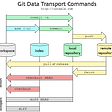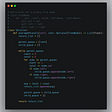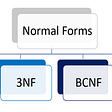# Maximum Depth of Binary Tree — Day 37(Python)

Today’s question is based on Trees. It tests your understanding of trees and recursion. It is an “Easy” tagged question in Leetcode. Let us look into the question.

104. Maximum Depth of Binary Tree

Given the `root` of a binary tree, return its maximum depth.

A binary tree’s maximum depth is the number of nodes along the longest path from the root node down to the farthest leaf node.

Example 1:

`Input: root = [3,9,20,null,null,15,7]Output: 3`

Example 2:

`Input: root = [1,null,2]Output: 2`

Example 3:

`Input: root = []Output: 0`

Example 4:

`Input: root = Output: 1`

Constraints:

• The number of nodes in the tree is in the range `[0, 104]`.
• `-100 <= Node.val <= 100`

The question requires us to find the maximum depth of the tree, which means we have to find the height of the tree. To find the height of the tree we would have to start from the root node and go until the leaf node. There can be multiple leaf nodes, we need to find the maximum among the recorded heights. We will be using recursion to solve this problem.

Before we use recursion, we need to understand what needs to be our base condition.

When the root is None, we should return 0.

Okay, now let's look at the code snippet.

`class Solution:    def maxDepth(self, root: TreeNode) -> int:        if root == None:            return 0        returnmax(self.maxDepth(root.left),self.maxDepth(root.right))+1`

Complexity analysis.

Time Complexity

We are visiting each node once and hence the time complexity is O(N) where N is the number of nodes in the Tree.

Space Complexity.

We are visiting each node recursively, hence the space complexity is O(N).

I would love to hear your feedback about my posts. Do let me know if you have any comments or feedback.

--

--

--

## More from Annamariya Tharayil

Software Engineer. Find me @ www.linkedin.com/in/annamariya-jt

Love podcasts or audiobooks? Learn on the go with our new app.

## Conversion Between Array and ArrayList in Java## Hacktoberfest 2021 — Week 1## Dev > Command Line > Git## What happens when you type gcc main.c## Caching function calls in Python 🐍## 5 Takeaways From Building a No-Code Website## Annamariya Tharayil

Software Engineer. Find me @ www.linkedin.com/in/annamariya-jt

## Jump Game: Leetcode Medium — Blind 75 (Greedy)## LeetCode Patterns Adventure 20— Average of Levels in Binary Tree## How to reverse integer using modulus operator(Python)## Database Normalization